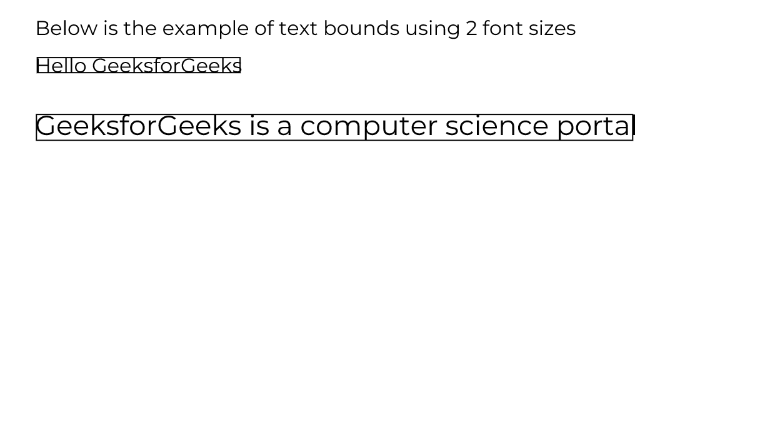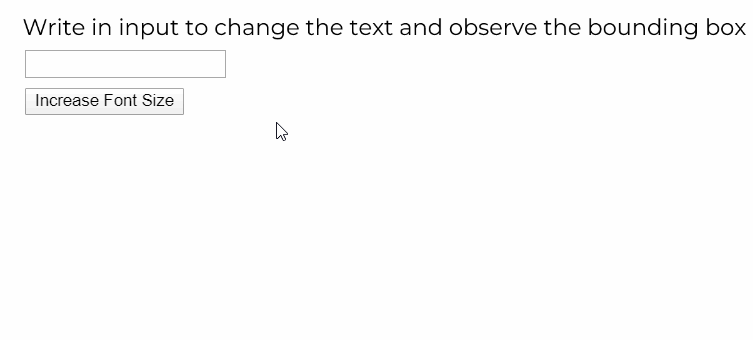Related Articles

# p5.js | p5.Font textBounds() Method

• Last Updated : 29 May, 2020

The textBounds() method of p5.Font in p5.js is to return a tight bounding box for the given string using the font it is used upon. This method supports only single lines.
Syntax:

```textBounds( line, x, y, [fontSize], [options] )
```

Parameters: This function accepts five parameters as mentioned above and described below:

• line: It is a String which denotes the line of text for which the bounding box has to be found.
• x: It is a Number which denotes the x-position.
• y: It is a Number which denotes the y-position .
• fontSize: It is a Number which denotes the font size to be used. The default value is 12. It is an optional parameter.
• options: It is an Object which can be used to specify opentype options. Opentype fonts contain options like alignment and baseline options. The default values are “LEFT” and “alphabetic”. It is an optional parameter.

The examples below illustrates the textBounds() function in p5.js:
Example 1:

## javascript

 `let inputElem;``let currFont;`` ` `function` `preload() {``  ``currFont = loadFont(``"fonts/Montserrat.otf"``);``}`` ` `function` `setup() {``  ``createCanvas(600, 300);`` ` `  ``textFont(currFont);``}`` ` `function` `draw() {``  ``clear();``  ``textSize(16);``  ``let text1 = ``"Hello GeeksforGeeks"``;``  ``let text2 = ``"GeeksforGeeks is a computer science portal"``;``  ``text(``"Below is the example of text bounds using 2 font sizes"``, 20, 20);`` ` `  ``// Set new font size``  ``let fontSizeSmall = 16;``  ``textSize(fontSizeSmall);``  ``// Get the bounding box dimensions``  ``let bounding_box = currFont.textBounds(text1, 20, 50, fontSizeSmall);``  ``// Draw the bounding box``  ``fill(255);``  ``stroke(0);``  ``rect(bounding_box.x, bounding_box.y, bounding_box.w, bounding_box.h);``  ``fill(0);``  ``noStroke();``  ``// Show the text``  ``text(text1, 20, 50);`` ` `  ``// Set new font size``  ``let fontSizeLarge = 22;``  ``textSize(fontSizeLarge);``  ``// Get the bounding box dimensions``  ``let bounding_box2 = currFont.textBounds(text2, 20, 100, fontSizeLarge);``  ``// Draw the bounding box``  ``fill(255);``  ``stroke(0);``  ``rect(bounding_box2.x, bounding_box2.y, bounding_box2.w, bounding_box2.h);``  ``fill(0);``  ``noStroke();`` ` `  ``text(text2, 20, 100);``}`

Output:Example 2:

## javascript

 `let inputElem;``let currfontSize = 28;``let currFont;`` ` `function` `preload() {``  ``currFont = loadFont(``"fonts/Montserrat.otf"``);``}`` ` `function` `setup() {``  ``createCanvas(600, 300);`` ` `  ``// Create button to increase font size``  ``let fontBtn = createButton(``"Increase Font Size"``);``  ``fontBtn.mouseClicked(() => {``    ``currfontSize += 2;``  ``});``  ``fontBtn.position(20, 70);`` ` `  ``// Create input box``  ``inputElem = createInput(``""``);``  ``inputElem.position(20, 40);`` ` `  ``textFont(currFont, currfontSize);``}`` ` `function` `draw() {``  ``clear();``  ``textSize(18);``  ``text(``    ``"Write in input to change the text and observe the bounding box"``,``    ``10,``    ``20``  ``);`` ` `  ``// Display text and line if input not empty``  ``let enteredText = inputElem.value();``  ``if` `(enteredText != ``""``) {``    ``// Get the bounding box dimensions``    ``let bounding_box = currFont.textBounds(enteredText, 20, 150, currfontSize);`` ` `    ``// Show the properites of the boundig box``    ``text(``"Box Position X: "` `+ bounding_box.x, 20, 180);``    ``text(``"Box Position Y: "` `+ bounding_box.y, 20, 200);``    ``text(``"Box Height: "` `+ bounding_box.h, 20, 220);``    ``text(``"Box Width: "` `+ bounding_box.w, 20, 240);`` ` `    ``textSize(currfontSize);`` ` `    ``// Set properties for drawing the box``    ``fill(255);``    ``stroke(0);`` ` `    ``// Draw the bounding box``    ``rect(bounding_box.x, bounding_box.y, bounding_box.w, bounding_box.h);``    ``fill(0);``    ``noStroke();`` ` `    ``// Show the entered text inside the box``    ``text(enteredText, 20, 150);``  ``}``}`

Output:Online editor: https://editor.p5js.org/
Environment Setup: https://www.geeksforgeeks.org/p5-js-soundfile-object-installation-and-methods/
Reference: https://p5js.org/reference/#/p5.Font/textBounds

Hey geek! The constant emerging technologies in the world of web development always keeps the excitement for this subject through the roof. But before you tackle the big projects, we suggest you start by learning the basics. Kickstart your web development journey by learning JS concepts with our JavaScript Course. Now at it’s lowest price ever!

My Personal Notes arrow_drop_up